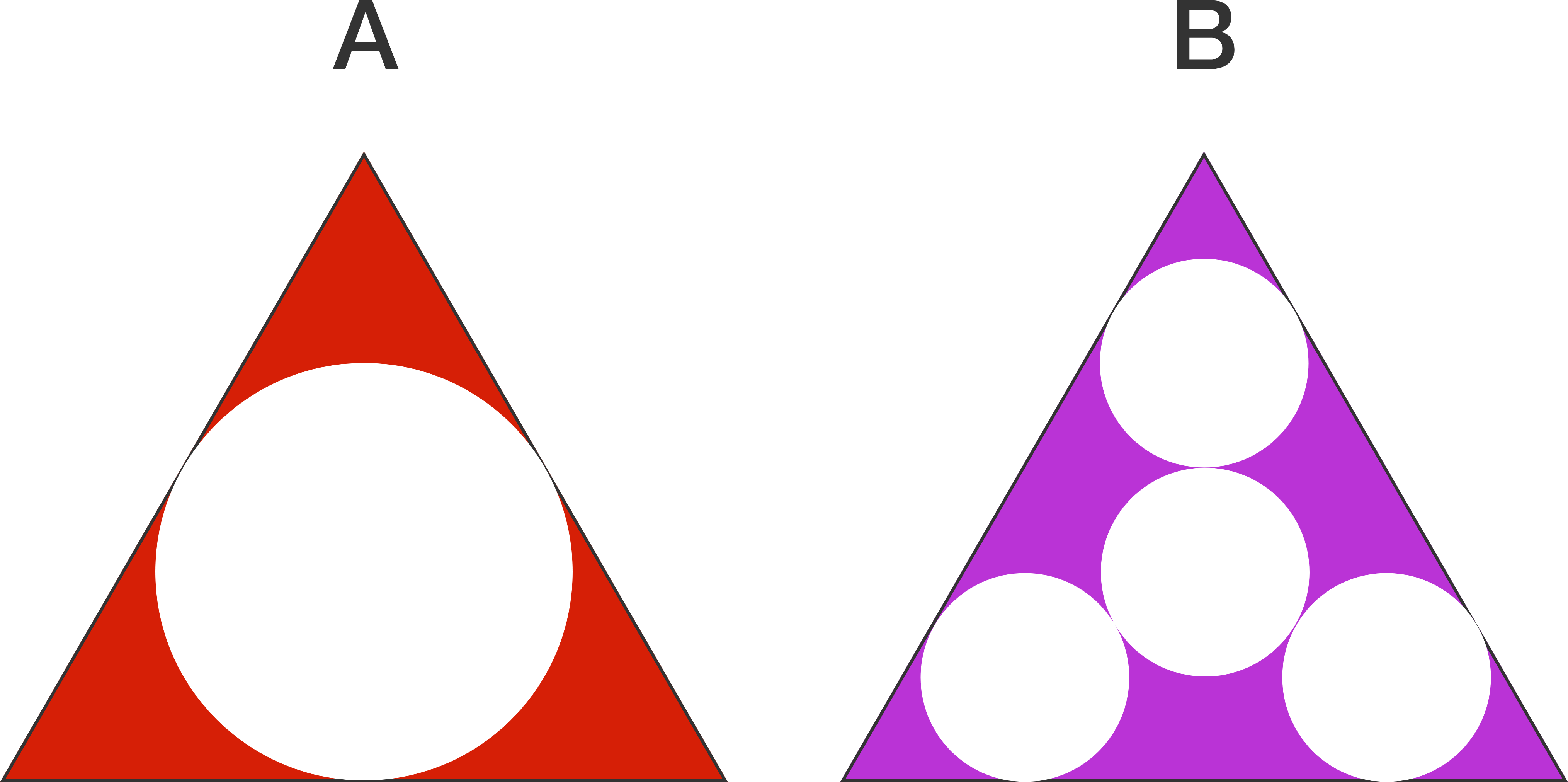# 37 of 100: Carved Triangles

Geometry Level 2If the two triangles above are congruent, equilateral triangles, which is greater, the total area of the three $\color{#D61F06}{\text{red}}$ regions or the total area of the six $\color{#69047E}{\text{purple}}$ regions?The circle in figure A is tangent to all sides of the triangle. The center circle of figure B is tangent to the other three circles, which are themselves tangent to the sides of the triangle; those four circles are all congruent.

×

Problem Loading...

Note Loading...

Set Loading...